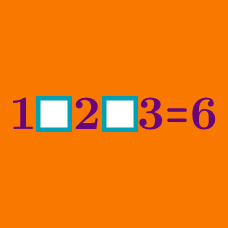Logic

# Addition and subtraction only - Operator Search

$1 \; \square \; 2 \; \square \; 4 \; \square \; 8 < 0$

Which of the following series of signs are appropriate for the three boxes above?

If you fill in the blanks with $+$ and/or $-$ only, how many $+$ do you need to use to make this equation true?

$2 \; \square \; 5 \; \square \; 6 \; \square \; 7 = 10$

$1 \; \square \; 1 \; \square \; 1 \; \square \; 1$

If we fill in the above blanks using only the operators $+$ and/or $-$, which of the following $\color{#D61F06} { \text{cannot} }$ be a resultant number?

$9 \; \square \; 7 \; \square \; 5 \; \square \; 3$

If we fill in the above blanks using only the operators $+$ and/or $-$, the resultant number will always be an $\text{\_\_\_\_\_\_\_\_\_\_}$.

$2 \; \square \; 3 \; \square \; 3\; \square \; 6 = 2$

Which of the following series of signs are $\color{#D61F06}{\text{not}}$ appropriate for the three boxes above?

×